# tsne

t-Distributed Stochastic Neighbor Embedding

## Syntax

``Y = tsne(X)``
``Y = tsne(X,Name,Value)``
``````[Y,loss] = tsne(___)``````

## Description

example

````Y = tsne(X)` returns a matrix of two-dimensional embeddings of the high-dimensional rows of `X`.```

example

````Y = tsne(X,Name,Value)` modifies the embeddings using options specified by one or more name-value pair arguments.```

example

``````[Y,loss] = tsne(___)```, for any input arguments, also returns the Kullback-Leibler divergence between the joint distributions that model the data `X` and the embedding `Y`.```

## Examples

collapse all

The Fisher iris data set has four-dimensional measurements of irises, and corresponding classification into species. Visualize this data by reducing the dimension using `tsne`.

```load fisheriris rng default % for reproducibility Y = tsne(meas); gscatter(Y(:,1),Y(:,2),species)```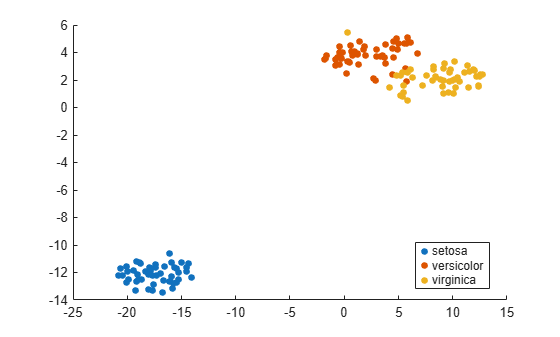Use various distance metrics to try to obtain a better separation between species in the Fisher iris data.

```load fisheriris rng('default') % for reproducibility Y = tsne(meas,'Algorithm','exact','Distance','mahalanobis'); subplot(2,2,1) gscatter(Y(:,1),Y(:,2),species) title('Mahalanobis') rng('default') % for fair comparison Y = tsne(meas,'Algorithm','exact','Distance','cosine'); subplot(2,2,2) gscatter(Y(:,1),Y(:,2),species) title('Cosine') rng('default') % for fair comparison Y = tsne(meas,'Algorithm','exact','Distance','chebychev'); subplot(2,2,3) gscatter(Y(:,1),Y(:,2),species) title('Chebychev') rng('default') % for fair comparison Y = tsne(meas,'Algorithm','exact','Distance','euclidean'); subplot(2,2,4) gscatter(Y(:,1),Y(:,2),species) title('Euclidean')```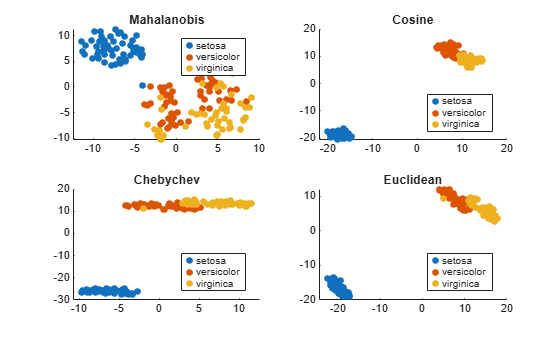In this case, the cosine, Chebychev, and Euclidean distance metrics give reasonably good separation of clusters. But the Mahalanobis distance metric does not give a good separation.

`tsne` removes input data rows that contain any `NaN` entries. Therefore, you must remove any such rows from your classification data before plotting.

For example, change a few random entries in the Fisher iris data to `NaN`.

```load fisheriris rng default % for reproducibility meas(rand(size(meas)) < 0.05) = NaN;```

Embed the four-dimensional data into two dimensions using `tsne`.

`Y = tsne(meas,'Algorithm','exact');`
```Warning: Rows with NaN missing values in X or 'InitialY' values are removed. ```

Determine how many rows were eliminated from the embedding.

`length(species)-length(Y)`
```ans = 22 ```

Prepare to plot the result by locating the rows of `meas` that have no `NaN` values.

`goodrows = not(any(isnan(meas),2));`

Plot the results using only the rows of `species` that correspond to rows of `meas` with no `NaN` values.

`gscatter(Y(:,1),Y(:,2),species(goodrows))`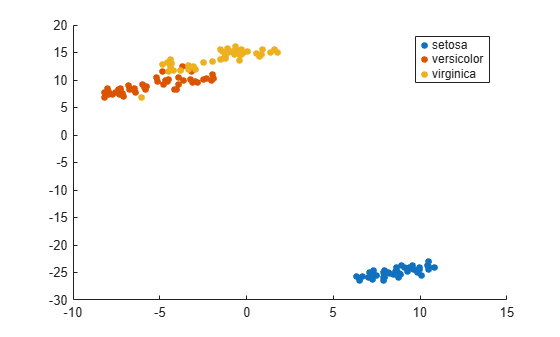Find both 2-D and 3-D embeddings of the Fisher iris data, and compare the loss for each embedding. It is likely that the loss is lower for a 3-D embedding, because this embedding has more freedom to match the original data.

```load fisheriris rng default % for reproducibility [Y,loss] = tsne(meas,'Algorithm','exact'); rng default % for fair comparison [Y2,loss2] = tsne(meas,'Algorithm','exact','NumDimensions',3); fprintf('2-D embedding has loss %g, and 3-D embedding has loss %g.\n',loss,loss2)```
```2-D embedding has loss 0.124191, and 3-D embedding has loss 0.0990884. ```

As expected, the 3-D embedding has lower loss.

View the embeddings. Use RGB colors `[1 0 0]`, `[0 1 0]`, and `[0 0 1]`.

For the 3-D plot, convert the species to numeric values using the `categorical` command, then convert the numeric values to RGB colors using the `sparse` function as follows. If `v` is a vector of positive integers 1, 2, or 3, corresponding to the species data, then the command

`sparse(1:numel(v),v,ones(size(v)))`

is a sparse matrix whose rows are the RGB colors of the species.

```gscatter(Y(:,1),Y(:,2),species,eye(3)) title('2-D Embedding')```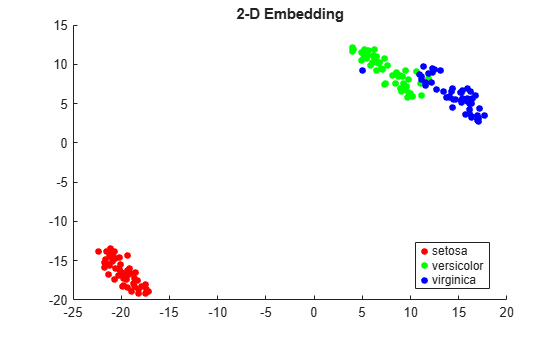```figure v = double(categorical(species)); c = full(sparse(1:numel(v),v,ones(size(v)),numel(v),3)); scatter3(Y2(:,1),Y2(:,2),Y2(:,3),15,c,'filled') title('3-D Embedding') view(-50,8)```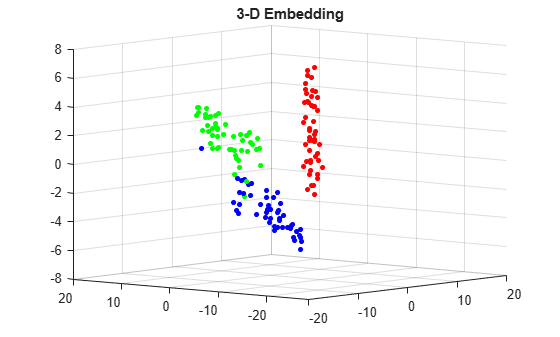## Input Arguments

collapse all

Data points, specified as an `n`-by-`m` matrix, where each row is one `m`-dimensional point.

`tsne` removes rows of `X` that contain any `NaN` values before creating an embedding. See Plot Results with NaN Input Data.

Data Types: `single` | `double`

### Name-Value Arguments

Specify optional comma-separated pairs of `Name,Value` arguments. `Name` is the argument name and `Value` is the corresponding value. `Name` must appear inside quotes. You can specify several name and value pair arguments in any order as `Name1,Value1,...,NameN,ValueN`.

Example: ```Y = tsne(X,'Algorithm','Exact','NumPCAComponents',50)```
Algorithm Control

collapse all

`tsne` algorithm, specified as `'barneshut'` or `'exact'`. The `'exact'` algorithm optimizes the Kullback-Leibler divergence of distributions between the original space and the embedded space. The `'barneshut'` algorithm performs an approximate optimization that is faster and uses less memory when the number of data rows is large.

Note

For the `'barneshut'` algorithm, `tsne` uses `knnsearch` to find the nearest neighbors.

Example: `'exact'`

Distance metric, specified by one of the following. For definitions of the distance metrics, see `pdist`.

• `'euclidean'` — Euclidean distance.

• `'seuclidean'` — Standardized Euclidean distance. Each coordinate difference between rows in `X` and the query matrix is scaled by dividing by the corresponding element of the standard deviation computed from `S = std(X,'omitnan')`.

• `'cityblock'` — City block distance.

• `'chebychev'` — Chebychev distance, which is the maximum coordinate difference.

• `'minkowski'` — Minkowski distance with exponent 2. This is the same as Euclidean distance.

• `'mahalanobis'` — Mahalanobis distance, computed using the positive definite covariance matrix `cov(X,'omitrows')`.

• `'cosine'` — 1 minus the cosine of the included angle between observations (treated as vectors).

• `'correlation'` — One minus the sample linear correlation between observations (treated as sequences of values).

• `'spearman'` — One minus the sample Spearman's rank correlation between observations (treated as sequences of values).

• `'hamming'` — Hamming distance, which is the percentage of coordinates that differ.

• `'jaccard'` — One minus the Jaccard coefficient, which is the percentage of nonzero coordinates that differ.

• custom distance function — A distance function specified using `@` (for example, `@distfun`). For details, see More About.

In all cases, `tsne` uses squared pairwise distances to calculate the Gaussian kernel in the joint distribution of `X`.

Example: `'mahalanobis'`

Size of natural clusters in data, specified as a scalar value `1` or greater.

A large exaggeration makes `tsne` learn larger joint probabilities of `Y` and creates relatively more space between clusters in `Y`. `tsne` uses exaggeration in the first 99 optimization iterations.

If the value of Kullback-Leibler divergence increases in the early stage of the optimization, try reducing the exaggeration. See tsne Settings.

Example: `10`

Data Types: `single` | `double`

Dimension of the output `Y`, specified as a positive integer. Generally, set `NumDimensions` to `2` or `3`.

Example: `3`

Data Types: `single` | `double`

PCA dimension reduction, specified as a nonnegative integer. Before `tsne` embeds the high-dimensional data, it first reduces the dimensionality of the data to `NumPCAComponents` using the `pca` function. When `NumPCAComponents` is `0`, `tsne` does not use PCA.

Example: `50`

Data Types: `single` | `double`

Effective number of local neighbors of each point, specified as a positive scalar. See t-SNE Algorithm.

Larger perplexity causes `tsne` to use more points as nearest neighbors. Use a larger value of `Perplexity` for a large dataset. Typical `Perplexity` values are from `5` to `50`. In the Barnes-Hut algorithm, `tsne` uses `min(3*Perplexity,N-1)` as the number of nearest neighbors. See tsne Settings.

Example: `10`

Data Types: `single` | `double`

Normalize input data, specified as `false` or `true`. When `true`, `tsne` centers and scales `X` by dividing the columns by their standard deviations.

When features in `X` are on different scales, set `'Standardize'` to `true`. Do this because the learning process is based on nearest neighbors, so features with large scales can override the contribution of features with small scales.

Example: `true`

Data Types: `logical`

Optimization Control

collapse all

Initial embedded points, specified as an `n`-by-`NumDimensions` real matrix, where `n` is the number of rows of `X`. The `tsne` optimization algorithm uses these points as initial values.

Data Types: `single` | `double`

Learning rate for optimization process, specified as a positive scalar. Typically, set values from `100` through `1000`.

When `LearnRate` is too small, `tsne` can converge to a poor local minimum. When `LearnRate` is too large, the optimization can initially have the Kullback-Leibler divergence increase rather than decrease. See tsne Settings.

Example: `1000`

Data Types: `single` | `double`

Iterative display frequency, specified as a positive integer. When the `Verbose` name-value pair is not `0`, `tsne` returns iterative display after every `NumPrint` iterations. If the `Options` name-value pair contains a nonempty `'OutputFcn'` entry, then output functions run after every `NumPrint` iterations.

Example: `20`

Data Types: `single` | `double`

Optimization options, specified as a structure containing the fields `'MaxIter'`, `'OutputFcn'`, and `'TolFun'`. Create `'Options'` using `statset` or `struct`.

• `'MaxIter'` — Positive integer specifying the maximum number of optimization iterations. Default: `1000`.

• `'OutputFcn'` — Function handle or cell array of function handles specifying one or more functions to call after every `NumPrint` optimization iterations. For syntax details, see t-SNE Output Function. Default: `[]`.

• `'TolFun'` — Stopping criterion for the optimization. The optimization exits when the norm of the gradient of the Kullback-Leibler divergence is less than `'TolFun'`. Default: `1e-10`.

Example: `options = statset('MaxIter',500)`

Data Types: `struct`

Barnes-Hut tradeoff parameter, specified as a scalar from 0 through 1. Higher values give a faster but less accurate optimization. Applies only when `Algorithm` is `'barneshut'`.

Example: `0.1`

Data Types: `single` | `double`

Iterative display, specified as `0`, `1`, or `2`. When `Verbose` is not `0`, `tsne` prints a summary table of the Kullback-Leibler divergence and the norm of its gradient every `NumPrint` iterations.

When `Verbose` is `2`, `tsne` also prints the variances of Gaussian kernels. `tsne` uses these kernels in its computation of the joint probability of `X`. If you see a large difference in the scales of the minimum and maximum variances, you can sometimes get more suitable results by rescaling `X`.

Example: `2`

Data Types: `single` | `double`

## Output Arguments

collapse all

Embedded points, returned as an `n`-by-`NumDimensions` matrix. Each row represents one embedded point. `n` is the number of rows of data `X` that do not contain any `NaN` entries. See Plot Results with NaN Input Data.

Kullback-Leibler divergence between modeled input and output distributions, returned as a nonnegative scalar. For details, see t-SNE Algorithm.

collapse all

### Custom Distance Function

The syntax of a custom distance function is as follows.

`function D2 = distfun(ZI,ZJ)`

`tsne` passes `ZI` and `ZJ` to your function, and your function computes the distance.

• `ZI` is a 1-by-n vector containing a single row from `X` or `Y`.

• `ZJ` is an m-by-n matrix containing multiple rows of `X` or `Y`.

Your function returns `D2`, which is an m-by-1 vector of distances. The jth element of `D2` is the distance between the observations `ZI` and `ZJ(j,:)`.

Tip

If your data are not sparse, then usually the built-in distance functions are faster than a function handle.

## Algorithms

`tsne` constructs a set of embedded points in a low-dimensional space whose relative similarities mimic those of the original high-dimensional points. The embedded points show the clustering in the original data.

Roughly, the algorithm models the original points as coming from a Gaussian distribution, and the embedded points as coming from a Student’s t distribution. The algorithm tries to minimize the Kullback-Leibler divergence between these two distributions by moving the embedded points.

For details, see t-SNE.